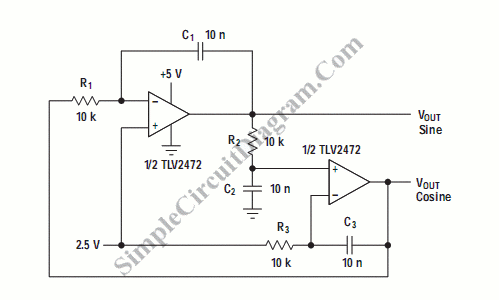# Quadrature Sinewave Oscillator

Quadrature oscillator is another type of phase shift oscillator. This circuit produce two sine wave signal. One of them is shifted 90 degree from the other signal. The output that shifted 90 degree is called cosine signal and the other is called sine circuit has two output. Here is the circuit :This circuit has three phase shifter sections. Each section is set to generate 90 degree phase shift. The frequency f would be equal to 1/2[pi]RC because R3C3=R2C2=R1C1. The value of the components might variate within their tolerance because when this circuit is tested, it generate 1.65 kHz rather than calculated 1.59 kHz. [Schematic diagram source: Analog Application Journal, August 2000]